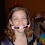## Tuesday, August 12, 2008

### First Step is To Assume No Friction

At the same time a block of iron and an equally heavy iron sphere are released from the top of a slope. Assume that the friction between the slope has a negligible effect on the velocity of the block and the sphere. Which of the two will be down first?

1.The block. A fraction (2/7, in the case of the sphere, I believe) of the potential energy will go into kinetic energy of rotation. However, all the potential energy goes into "translational" kinetic energy, and none is lost to rotation.

2.I may be wrong, but wouldn't the sphere only rotate in proportion to the amount of friction (if there's no friction it will also slide), so the energy used in rotating the sphere is a proportion of the energy lost through friction and more energy would be lost from the block due to the same coefficient of friction between iron and slope (larger surface area in contact with the slope, block cannot translate this deceleration into rotation, etc.). So unless there is no friction, the sphere will still travel down the slope faster. So assuming that there is no friction the sphere also slides. As the sphere is more aerodynamic it should reach the bottom first, unless we're in a vacuum in which case they would both reach the bottom at the same time.

3.That's a very good question. If there is no friction, would the ball start rolling, or would it slide along like the block.

I think, and it's been way too long since my basic physics courses, the ball would start rolling since it's center of gravity would move it. But I'm not sure given there's no friction to 'push it back.'

Is anyone a resident expert on such matters and want to chime in?

If you assume the ball will roll, then Abe is right and the ball will reach the bottom after the block since some of the energy is being moved into rotation.

I don't think (even if the ball didn't start rolling) the ball could beat the block down, since we're assuming no friction. There would be no drag by the air, which was rich's argument. So, if the ball isn't rotating, then the block and ball would slide at the same rate, just as rich said.

4.If there was really no friction anywhere at all, then the ball would not roll, and the two bodies would arrive at the same time. "Static" friction (the friction of two bodies that aren't sliding against each other) is the thing that keeps the ball rolling. This force usually is considerably stronger than "kinetic friction" (friction of two bodies sliding against each other), which is the thing that would affect the sliding block. This is a common physics problem. When you said to assume friction is negligible, instead of assuming there is no friction, it seemed like what you were after is the rotational energy thing.

Anyway, the cool thing about problems like this, is that even objects of different shapes will roll at different speeds. It has to do only with the geometry. Hollow spheres, solid spheres, hollow cylinders, solid cylinders, etc., all roll down the slope at different speeds because of their geometries (specifically, how hard it is to "roll" them from their outer edge, and therefore how much energy has to go into it). I could go on about this, but you can look it up on wikipedia or something...

Leave your answer or, if you want to post a question of your own, send me an e-mail. Look in the about section to find my e-mail address. If it's new, I'll post it soon.

Please don't leave spam or 'Awesome blog, come visit mine' messages. I'll delete them soon after.

Enter your Email and join hundreds of others who get their Question of the Day sent right to their mailbox

The Lamplight Manor Puzz 3-D